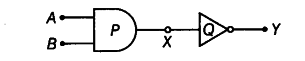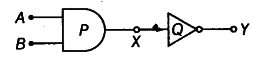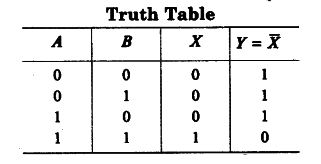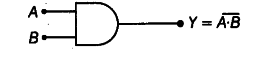# Identify the gates P and Q shown in the figure

Identify the gates P and Q shown in the figure. Write the truth table for the combination of gates as shown in figure below:Name the equivalent gate representing this circuit and write its logic symbol

Consider the given figure,P is AND gate and Q is a NOT gate.The equivalent gate representing this circuit is NAND gate. Its logic symbol is